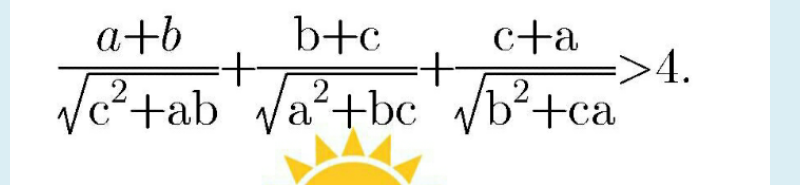# Doubt from InequlatiesBy Holder's Inequality

\displaystyle \sum \dfrac{a+b}{\sqrt{c^2+ab}} \sum \dfrac{a+b}{\sqrt{c^2+ab}} \sum (a+b)(c^2+ab) \ge (\sum (a+b))^3=8(a+b+c)^3

Hence it suffices to show that 8(a+b+c)^3 \ge 16 \sum (a+b)(c^2+ab)

or (a+b+c)^3 \ge 2 \sum (a+b)(c^2+ab) =4 \sum ab(a+b)

or \sum a^3+3 \sum ab(a+b)+6abc \ge 4 \sum ab(a+b)

or \sum a^3+6abc \ge \sum ab(a+b)

But by Schur's Inequality we have \sum a^3+3abc \ge \sum ab(a+b) and hence the required inequality is clearly true

4 Likes

I didnt really do much in this question as it is similar to IMO 2001 problem #2 which I remember the solution to

1 Like